Past Year Paper 2018 ( Electrical Engineering)

# Past Year Paper 2018 ( Electrical Engineering)

Test Description

## 31 Questions MCQ Test | Past Year Paper 2018 ( Electrical Engineering)

Past Year Paper 2018 ( Electrical Engineering) for Electrical Engineering (EE) 2022 is part of Electrical Engineering (EE) preparation. The Past Year Paper 2018 ( Electrical Engineering) questions and answers have been prepared according to the Electrical Engineering (EE) exam syllabus.The Past Year Paper 2018 ( Electrical Engineering) MCQs are made for Electrical Engineering (EE) 2022 Exam. Find important definitions, questions, notes, meanings, examples, exercises, MCQs and online tests for Past Year Paper 2018 ( Electrical Engineering) below.
Solutions of Past Year Paper 2018 ( Electrical Engineering) questions in English are available as part of our course for Electrical Engineering (EE) & Past Year Paper 2018 ( Electrical Engineering) solutions in Hindi for Electrical Engineering (EE) course. Download more important topics, notes, lectures and mock test series for Electrical Engineering (EE) Exam by signing up for free. Attempt Past Year Paper 2018 ( Electrical Engineering) | 31 questions in 180 minutes | Mock test for Electrical Engineering (EE) preparation | Free important questions MCQ to study for Electrical Engineering (EE) Exam | Download free PDF with solutions
 1 Crore+ students have signed up on EduRev. Have you?
Past Year Paper 2018 ( Electrical Engineering) - Question 1

### For what values of k given below is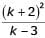an integer?

Detailed Solution for Past Year Paper 2018 ( Electrical Engineering) - Question 1

Sincemust be an integer, we can verify the options.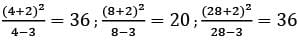So, option (c) Þ 4, 8, 28

Past Year Paper 2018 ( Electrical Engineering) - Question 2

### “Since you have gone off the _________, the _________ sand is likely to damage the car”. The words that best fill the blanks in the above sentence are

Detailed Solution for Past Year Paper 2018 ( Electrical Engineering) - Question 2

Going off the course – not following the intended route.
Coarse sand – harsh in texture

Past Year Paper 2018 ( Electrical Engineering) - Question 3

### The three roots of the equation f (x ) = 0 are x = {-2, 0, 3}. What are the three values of for which f (x - 3)= 0 ?

Detailed Solution for Past Year Paper 2018 ( Electrical Engineering) - Question 3

f(x) = 0 for x = {−2,0,3}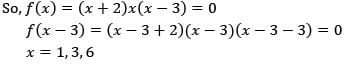Past Year Paper 2018 ( Electrical Engineering) - Question 4

Functions, F ( a, b) and G ( a, b) are defined as follows:
F (a, b) = ( a− b)2 and G ( a, b) = |a− b|, where |x| represents the absolute value of x. What would be the value of G ( F (1, 3) , G (1, 3)) ?

Detailed Solution for Past Year Paper 2018 ( Electrical Engineering) - Question 4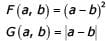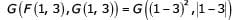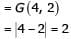Past Year Paper 2018 ( Electrical Engineering) - Question 5

“A common misconception among writers is that sentence structure mirrors thought; the more _________ the structure, the ted the ideas”.

Detailed Solution for Past Year Paper 2018 ( Electrical Engineering) - Question 5

Because the second half of the sentence illustrates the idea that “structure mirrors thought,” any word that fills the blank must be similar in meaning to “convoluted.” The two words that are similar to “convoluted” are “complicated” and “involved”, which produce sentences alike in meaning. “Fanciful,” while somewhat similar in meaning to “convoluted,” is not as similar to either “complicated” or “involved” as those words are to each other. The other answer choices are not similar in meaning to “convoluted,” and thus do not produce coherent sentences.
Thus the correct answer is complicated.

Past Year Paper 2018 ( Electrical Engineering) - Question 6

In a certain code AMCF is written as EQGJ and NKUF is written as ROYJ. How will DHLP be written in the code?

Detailed Solution for Past Year Paper 2018 ( Electrical Engineering) - Question 6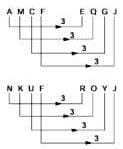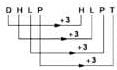Past Year Paper 2018 ( Electrical Engineering) - Question 7

An e-mail password must contain three characters. The password has to contain one numeral from 0 to 9, one upper case and one lower case character from the English alphabet. How many distinct passwords are possible?

Detailed Solution for Past Year Paper 2018 ( Electrical Engineering) - Question 7

Since, there are three positions which can be filled either by upper case letter, lower case letter or a number from 0 9 −
Hence, total choices are = 26 × 26 × 10 = 6760
3 characters can be arranged in 3! ways & hence total no of password can be = 3! × 6760 = 40560

Past Year Paper 2018 ( Electrical Engineering) - Question 8

A designer uses marbles of four different colours of his designs. The cost of each marble is the same, irrespective of the colour. The table below shows the percentage of marbles of each colour used in the current design. The cost of each marble increased by 25%. Therefore, the designer decided to reduce equal numbers of marbles of each colour to keep the total cost unchanged. What is the percentage of blue marbles in the new design?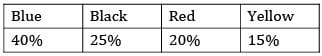Detailed Solution for Past Year Paper 2018 ( Electrical Engineering) - Question 8

Assume total number of marbles = 100
Blue marble = 40
Black marble = 25
Red marble = 20
Yellow marble = 15
Cost of 1 marble be Rs. 1
Cost of marbles increased = 25%
New cost of 1 marble = Rs. 1.25
Let x marbles are reduced from each type but the total cost must remain same i.e. Rs 100
Now,
1.25[(40 − x) + (25 − x) + (20 − x) + (15 − x)] = 100
125 − 5x = 100
25 = 5x
x = 5
So, now the numbers of different marbles are
Blue marble = 35
Black marble = 20
Red marble = 15
Yellow marble = 10
% blue marbles in new design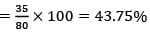Past Year Paper 2018 ( Electrical Engineering) - Question 9

P, Q, R and S crossed a lake in a boat that can hold a maximum of two persons, with only one set of oars. The following additional facts are available
i. The boat held two persons on each of the three forward trips across lake and one person on each of the two return trips.
ii. P is unable to row when someone else is in the boat.
iii. Q is unable to row with anyone else except R.
iv. Each person rowed for at least one trip.
v. Only one person can row during a trip.
Who rowed twice?

Detailed Solution for Past Year Paper 2018 ( Electrical Engineering) - Question 9

Since, P cannot row with anyone else so P must row while on return journey.
So, on first trip P & S leave in which S rows.
On the return trip P will row
On next trip P will leave with R and R will row & P will get down on other end.
Now R will return & row to pick Q up.
On the last trip Q & R will go in which Q will row the boat.
Hence, R rowed the boat twice

Past Year Paper 2018 ( Electrical Engineering) - Question 10

A class of twelve children has two more boys than girls. A group of three children are randomly picked from this class to accompany the teacher on a field trip. What is the probability that the group accompanying the teacher contains more girls than boys?

Detailed Solution for Past Year Paper 2018 ( Electrical Engineering) - Question 10

There are 7B and 5G
Through as question is stating 3 students are taken at random.
This can be a possible way to approach it.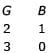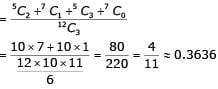Past Year Paper 2018 ( Electrical Engineering) - Question 11

Match the transfer functions of the second-order systems with the nature of the system given below.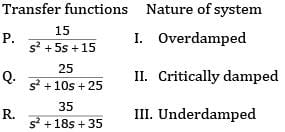Detailed Solution for Past Year Paper 2018 ( Electrical Engineering) - Question 11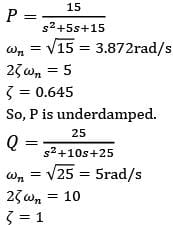So, Q is critically damped.
Observing all the options, option (c) is correct.

Past Year Paper 2018 ( Electrical Engineering) - Question 12

Consider a lossy transmission line with V1 and V2 as the sending and receiving end voltages, respectively. Z and X are the series impedance and reactance of the line, respectively. The steady-state stability limit for the transmission line will be

Detailed Solution for Past Year Paper 2018 ( Electrical Engineering) - Question 12

With only x :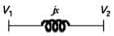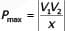With Lossy Tr. Line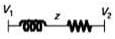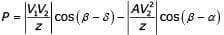∴ With Lossy Line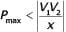Past Year Paper 2018 ( Electrical Engineering) - Question 13

Two wattmeter method is used for measurement of power in a balanced three-phase load supplied from a balanced three-phase system. If one of the wattmeter reads half of the other (both positive), then the power factor of the load is

Detailed Solution for Past Year Paper 2018 ( Electrical Engineering) - Question 13

In two Wattmeter method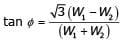Given :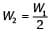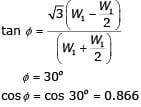Past Year Paper 2018 ( Electrical Engineering) - Question 14

A continuous-time input signal x (t ) is an eigen function of an LTI system, if the output is

Detailed Solution for Past Year Paper 2018 ( Electrical Engineering) - Question 14

If the output signal is a scalar multiple of input signal, the signal is refereed as an eigen function (or characteristic function) and the multiplier is referred as an eigen value (or characteristic value).
If x(t) is the eigen function and k is the eigen value, then output, y(t) = kx(t).
Hence, the correct option is (A).

Past Year Paper 2018 ( Electrical Engineering) - Question 15

In the logic circuit shown in the figure, Y is given by

Detailed Solution for Past Year Paper 2018 ( Electrical Engineering) - Question 15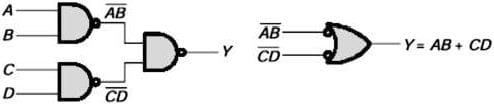*Answer can only contain numeric values
Past Year Paper 2018 ( Electrical Engineering) - Question 16

The value of the directional derivative of the function φ( x, y, z ) = xy2 + yz2 + zx2 at the point (2, -1, 1) in the direction of the vector p = i + 2 j +2k is

Detailed Solution for Past Year Paper 2018 ( Electrical Engineering) - Question 16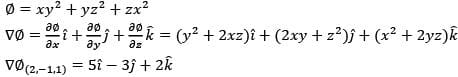The directional derivative of φ ( x, y, z ) at in (2, -1, 1) the direction of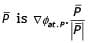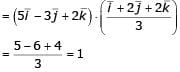*Answer can only contain numeric values
Past Year Paper 2018 ( Electrical Engineering) - Question 17

The waveform of the current drawn by a semi-converter from a sinusoidal AC voltage source is shown in the figure. If I0 = 20 A, the rms value of fundamental component of the current is __________ A (up to 2 decimal places).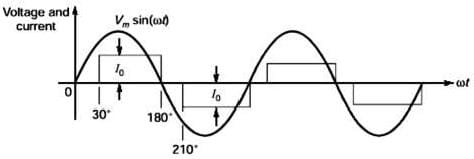Detailed Solution for Past Year Paper 2018 ( Electrical Engineering) - Question 17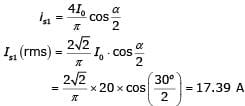*Answer can only contain numeric values
Past Year Paper 2018 ( Electrical Engineering) - Question 18

The series impedance matrix of a short three-phase transmission line in phase coordinates is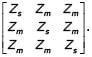If the positive sequence impedance is (1 + j10)Ω, and the zero sequence is (4 + j31)Ω, then the imaginary part of Zm (in W) is __________ (up to 2 decimal places).

Detailed Solution for Past Year Paper 2018 ( Electrical Engineering) - Question 18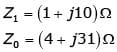We know,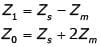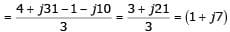The imaginary part of Zm is 7.00.

*Answer can only contain numeric values
Past Year Paper 2018 ( Electrical Engineering) - Question 19

In the two-port network shown, the h11 parameter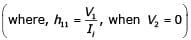in ohms is ________ (up to 2 decimal places).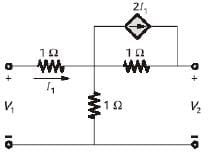Detailed Solution for Past Year Paper 2018 ( Electrical Engineering) - Question 19

To find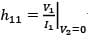the equivalent circuit is given below.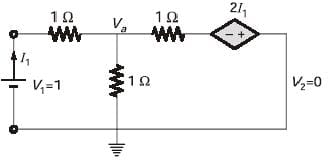By KCL,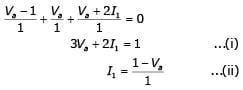Substitute equation (ii) in equation (i),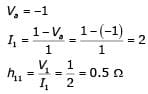Past Year Paper 2018 ( Electrical Engineering) - Question 20

The graph of a network has 8 nodes and 5 independent loops. The number of branches of the graph is

Detailed Solution for Past Year Paper 2018 ( Electrical Engineering) - Question 20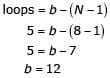Past Year Paper 2018 ( Electrical Engineering) - Question 21

In a salient pole synchronous motor, the developed reluctance torque attains the maximum value when the load angle in electrical degree is

Detailed Solution for Past Year Paper 2018 ( Electrical Engineering) - Question 21

Reluctance power is given by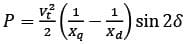(Vt is the line voltage)
So, reluctance torque is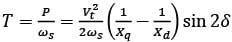So, torque is maximum when 2δ = 90°
δ = 45°

Past Year Paper 2018 ( Electrical Engineering) - Question 22

A single-phase fully controlled rectifier is supplying a load with an anti-parallel diode as shown in the figure. All switches and diodes are ideal. Which one of the following is true for instantaneous load voltage and current?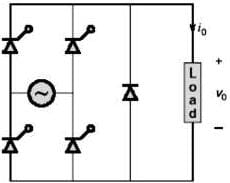Detailed Solution for Past Year Paper 2018 ( Electrical Engineering) - Question 22

Since, this converter has freewheeling diode, the output voltage cannot go negative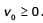Since, diodes and SCR are unidirectional current devices so current cannot reverse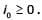*Answer can only contain numeric values
Past Year Paper 2018 ( Electrical Engineering) - Question 23

Let f be a real valued function of a real variable defined as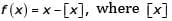denotes the largest integer less than or equal to x. The value of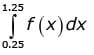is ________ (up to 2 decimal places).

Detailed Solution for Past Year Paper 2018 ( Electrical Engineering) - Question 23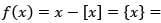fractional part of x Graph of fractional part of x is given below.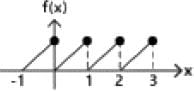It is periodic with period 1.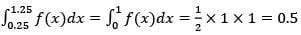Past Year Paper 2018 ( Electrical Engineering) - Question 24

A positive charge of 1 nC is placed at (0, 0, 0.2) where all dimensions are in meters. Consider the x-y plane to be a conducting ground plane. Take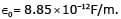The Z component of the E field at (0, 0, 0.1) is closed to

Detailed Solution for Past Year Paper 2018 ( Electrical Engineering) - Question 24

Due to infinite conducting plane, we have to consider an image charge symmetrically below plane. Since one charge is placed at (0, 0, 0.2), the image charge will be at (0, 0, -0.2)
Electric field at P will be sum of Field due to Q & -Q Net electric field at point due to a charge Q is given by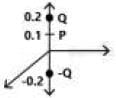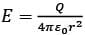So, electric field a point P is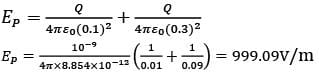Since, it is in downward direction so, Ep = −999.09V/m

*Answer can only contain numeric values
Past Year Paper 2018 ( Electrical Engineering) - Question 25

Consider a unity feedback system with forward transfer function given by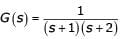The steady-state error in the output of the system for a unit-step input is __________ (upto 2 decimal places).

Detailed Solution for Past Year Paper 2018 ( Electrical Engineering) - Question 25

Steady-state error for type-0 and step input,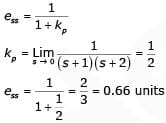*Answer can only contain numeric values
Past Year Paper 2018 ( Electrical Engineering) - Question 26

Consider a non-singular 2 * 2 square matrix A. If trace ( A) = 4 and trace ( A2 ) = 5, the determinant of the matrix A is ________ (up 1 decimal place).

Detailed Solution for Past Year Paper 2018 ( Electrical Engineering) - Question 26

A is 2 * 2 matrix
Let λ1 and λ2 be the eigen value of matrix A.
Since, sum of eigen values = trace of a matrix
Therefore, λ1 + λ2 = 4
And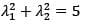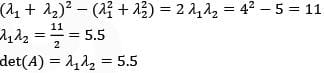*Answer can only contain numeric values
Past Year Paper 2018 ( Electrical Engineering) - Question 27

A 1000 * 1000 bus admittance matrix for an electric power system has 8000 non-zero elements. The minimum number of branches (transmission lines and transformers) in this system are ________ (upto 2 decimal places).

Detailed Solution for Past Year Paper 2018 ( Electrical Engineering) - Question 27

Number of buses = Number of diagonal elements = 1000
No. of non-zero elements = 8000
No. of non-zero off diagonal elements = 7000
No. of transmission lines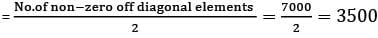Past Year Paper 2018 ( Electrical Engineering) - Question 28

In the figure, the voltages are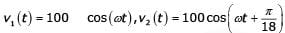and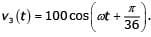The circuit is in sinusoidal steady-state, and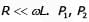and P3 are the average power outputs. Which one of the following statements is true?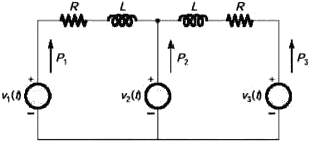Detailed Solution for Past Year Paper 2018 ( Electrical Engineering) - Question 28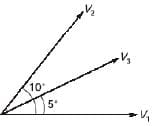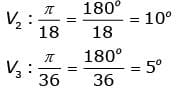So, current will flow from V2 towards V1 and V3.
Hence,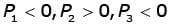Past Year Paper 2018 ( Electrical Engineering) - Question 29

The op-amp shown in the figure is ideal. The input impedance is given by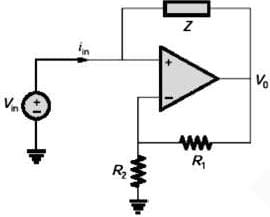Detailed Solution for Past Year Paper 2018 ( Electrical Engineering) - Question 29

According to virtual ground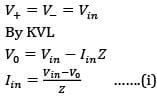Also by voltage division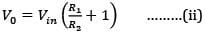Equation (ii) in equation (i)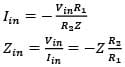Past Year Paper 2018 ( Electrical Engineering) - Question 30

The value of the integral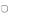in counter clockwise direction around a circle C of radius 1 with center at the point z =−2 is

Detailed Solution for Past Year Paper 2018 ( Electrical Engineering) - Question 30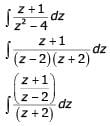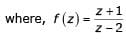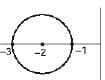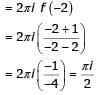*Answer can only contain numeric values
Past Year Paper 2018 ( Electrical Engineering) - Question 31

The positive, negative and zero sequence impedances of a 125 MVA, three-phase, 15.5 kV, star-grounded, 50 Hz generator are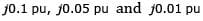respectively on the machine rating base. The machine is unloaded and working at the rated terminal voltage. If the grounding impedance of the generator is j0.01 pu then the magnitude of fault current for a b-phase to ground fault (in kA) is _______ (upto 2 decimal places).

Detailed Solution for Past Year Paper 2018 ( Electrical Engineering) - Question 31

For LG fault,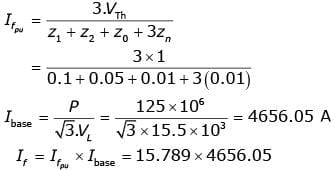= 73516.538 = 73.52kA

 Use Code STAYHOME200 and get INR 200 additional OFF Use Coupon Code
Information about Past Year Paper 2018 ( Electrical Engineering) Page
In this test you can find the Exam questions for Past Year Paper 2018 ( Electrical Engineering) solved & explained in the simplest way possible. Besides giving Questions and answers for Past Year Paper 2018 ( Electrical Engineering), EduRev gives you an ample number of Online tests for practice